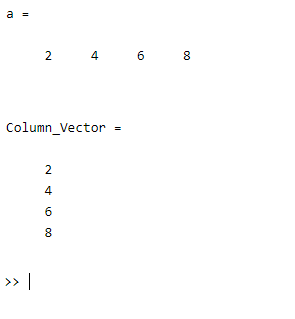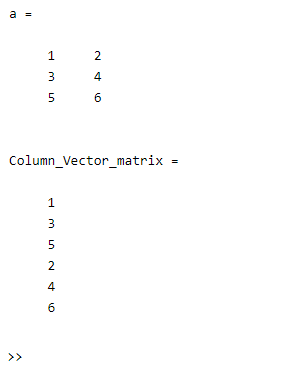Open in App
Not now

# Turn an Array into a Column Vector in MATLAB

• Last Updated : 04 Jul, 2021

Conversion of an Array into a Column Vector. This conversion can be done using a(:) operation. A(:) reshapes all elements of A into a single column vector. This has no effect if A is already a column vector.

Example 1

## Matlab

 `% MATLAB code for Conversion of an array ``% into a column vector``a = [2 4 6 8] ``% Initializing an array of some elements`` ` `% Converting above array into a column ``% vector using a(:) operation``Column_Vector = a(:)`

Output:Example 2

## Matlab

 `% MATLAB code for Conversion of an ``% array matrix into a column vector.``a = [1 2; 3 4; 5 6] ``% Initializing an array`` ` `% Converting above array matrix into a column ``% vector matrix using a(:) operation``Column_Vector_matrix = a(:)`

Output:My Personal Notes arrow_drop_up